##### Algebra II Workbook For Dummies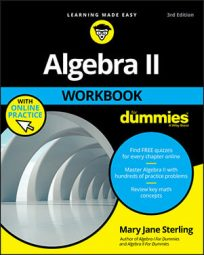Learning some algebraic rules for various exponents, radicals, laws, binomials, formulas, and equations will help you successfully study and solve problems in an Algebra II course. You should also be able to recognize formulas to find slope, slope-intercept, distance, and midpoint (which are formulas from geometry) to help you through Algebra II.

## Algebra: Rules of exponents

Exponents are shorthand for repeated multiplication. The rules for performing operations involving exponents allow you to change multiplication and division expressions with the same base into something simpler to work with. Remember that in xa, the x is the base and the a is the exponent.

Assume x ≠ 0: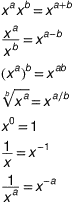## Linear equations: how to find slope, y-intercept, distance, midpoint

In algebra, linear equations means you’re dealing with straight lines. When you’re working with the xy-coordinate system, you can use the following formulas to find the slope, y-intercept, distance, and midpoint between two points.

Consider the two points (x1, y1) and (x2, y2):

Slope of the line through the points: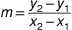Slope-intercept form of the line with y-intercept b: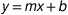Point-slope form of the line with slope m: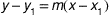Distance formula: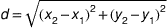Midpoint formula: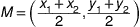## Rewrite absolute value equations as linear equations

To work with an absolute value equation in algebra, you first need to rewrite it as a linear equation. The same goes for an absolute value inequality, which you rewrite as a linear inequality.

When rewriting absolute value equations or inequalities, you drop the absolute value bars.

|ax + b| = c ax + b = c or ax + b = –c

|ax + b| > c → ax + b > c or ax + b > –c

|ax + b| < c →c < ax + b < c

## 9 number systems in algebra to know

A number system in algebra is a set of numbers — and different number systems are used to solve different types of algebra problems. Number systems include real numbers, natural numbers, whole numbers, integers, rational numbers, irrational numbers, even numbers, and odd numbers.

• Real numbers: Real numbers comprise the full spectrum of numbers. They cover the gamut and can take on any form — fractions or whole numbers, decimal points or no decimal points. The full range of real numbers includes decimals that can go on forever. Real numbers are different from imaginary or complex numbers.

• Natural numbers: A natural number is a number that comes naturally. What numbers did you first use? Remember someone asking, “How old are you?” You proudly held up four fingers and said, “Four!” Natural numbers are greater than zero but don’t include fractions: 1, 2, 3, 4, 5, 6, 7, and so on, into infinity. You use natural numbers to count items and to make lists.

• Whole numbers: Whole numbers are just all the natural numbers plus a zero: 0, 1, 2, 3, 4, 5, and so on, into infinity. They act like natural numbers and are used when whole amounts (no fractions) are required. Zero can also indicate none.

Algebraic problems often require you to round the answer to the nearest whole number. This makes perfect sense when the problem involves people, cars, animals, houses, or anything that shouldn’t be cut into pieces.

• Integers: Integers incorporate all the whole numbers and their opposites (or additive inverses of the whole numbers). Integers can be described as being positive and negative whole numbers and 0: . . . –3, –2, –1, 0, 1, 2, 3, . . . .

Integers are popular in algebra. When you solve a long, complicated problem and come up with an integer, you can be joyous because your answer is probably right. After all, it’s not a fraction! This doesn’t mean that answers in algebra can’t be fractions or decimals. It’s just that most textbooks and reference books try to stick with nice answers to increase the comfort level and avoid confusion.

• Rational numbers: Rational numbers are numbers that act rationally! In this case, acting rationally means that the decimal equivalent of the rational number behaves. The decimal ends somewhere, or it has a repeating pattern to it. That’s what constitutes “behaving.” Some examples of rational numbers with decimals that terminate include 2, 3.4, 5.77623, and –4.5.

Some examples of rational numbers with decimals that repeat the same pattern include the following: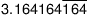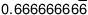(The horizontal bar over the 164 and the 6 lets you know that these numbers repeat forever.) In all cases, rational numbers can be written as a fraction. They all have a fraction that they are equal to.

• Irrational numbers: Irrational numbers are real numbers that are not rational numbers. An irrational number cannot be written as a fraction, and decimal values for irrationals never end and never have a nice pattern to them. For example, pi, with its never-ending decimal places, is irrational.

• Imaginary/complex numbers: A number that isn’t real can be imaginary or complex. An imaginary number contains some multiple of i, which is the following: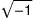For example, 2 + 3i is a complex number.

• Even numbers: An even number is one that divides evenly by 2, such as 2, 4, 18, and 352.

• Odd numbers: An odd number is one that does not divide evenly by 2, such as 1, 3, 27, and 485.

## Algebra II: What is the binomial theorem?

A binomial is a mathematical expression that has two terms. In algebra, people frequently raise binomials to powers to complete computations. The binomial theorem says that if a and b are real numbers and n is a positive integer, then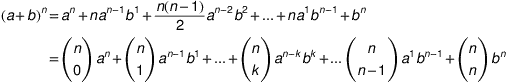You can see the rule here, in the second line, in terms of the coefficients that are created using combinations. The powers on a start with n and decrease until the power is zero in the last term. That’s why you don’t see an a in the last term — it’s a0, which is really a 1. The powers on b increase from b0 until the last term, where it’s bn. Notice that the power of b matches k in the combination.

## Use the properties of proportions to simplify fractions

In algebra, the properties of proportions come in handy when solving equations involving fractions. When you can, change an algebraic equation with fractions in it to a proportion for easy solving.

If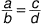then the following are all true: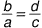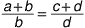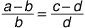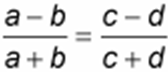A proportion is an equation involving two ratios (fractions) set equal to each other. The following equation is a proportion: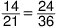Both fractions in that proportion reduce to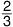so it’s fairly easy to see how this statement is true.

Proportions have some interesting, helpful, and easy-to-use properties. For example, in the following proportion,the cross-products are equal: a d = b c.

The reciprocals are equal (you can flip the fractions):You can reduce the fractions vertically or horizontally: You can divide out factors that are common to both numerators or both denominators or the left fraction or the right fraction. (You can’t, however, divide out a factor from the numerator of one fraction and the denominator of the other.)

## Algebra II: Raise binomials to a power

A binomial is a mathematical expression that has two terms. In algebra, people frequently raise binomials to powers in order to solve equations. Here are some examples:

(a + b)0 = 1

(a + b)1 = a + b

(a + b)2 = a2 + 2ab + b2

(a + b)3 = a3 + 3a2b + 3ab2 + b3

(a + b)4 = a4 + 4a3b + 6a2b2 + 4ab3 + b4

(a + b)5 = a5 + 5a4b + 10a3b2 + 10a2b3 + 5ab4 + b5